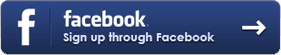Xphirience Bliss Encoded Sound Meditation

Here is yet another type of sound meditation. It is based on a mathematical principle that appears frequently in art and nature. The principle is called the Golden Ratio.

The golden ratio is described in Wikipedia as

In mathematics and the arts, two quantities are in the golden ratio (φ) if the ratio of the sum of the quantities to the larger quantity is equal to the ratio of the larger quantity to the smaller one. The figure on the right illustrates the geometric relationship.